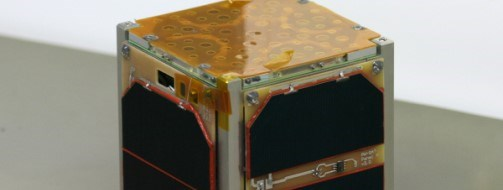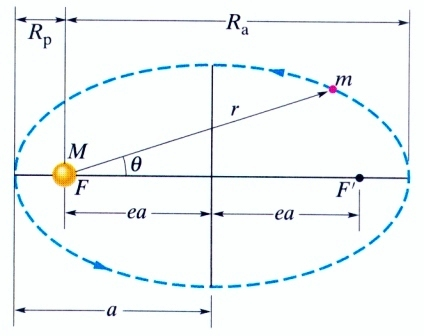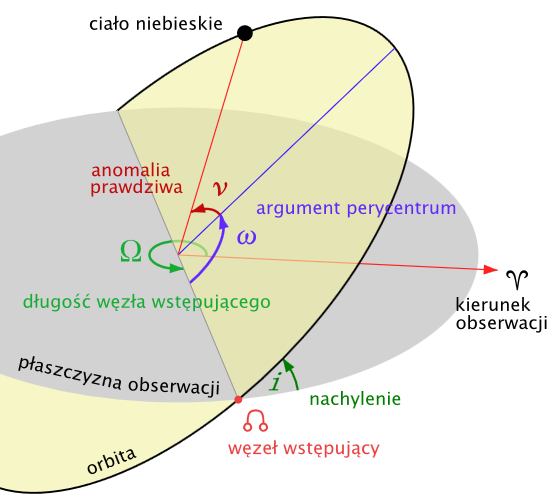13th of February 2014 is the second anniversary of launch of the first Polish student-made satellite PW-Sat. Currently Students’ Space Association on Warsaw University  of Technology is working on another satellite – PW-Sat2. Despite the lost communication with the satellite, the team has not forgotten about its predecessor and regularly monitors its status. Orbital parameters are analyzed to determine the satellites altitude, the time it takes to fully rotate around Earth as well as shape and size of the orbit. For this purpose, we use the data provided by NORAD (North American Aerospace called Defense Command) – the entity controlling the air space over North America and the space around the Earth.The data is stored in TLE format (called Two-Line Elements) in the form of two rows of orbit parameters of artificial satellites of the Earth. This system is commonly used by NORAD and NASA to track objects orbiting the Earth. Data about thousands of objects in space is generally available on the internet. To get the data file one should go to http://www.celestrak.com/NORAD/elements/ website and fill out the form by entering your personal data, the satellite number in the NORAD directory and the wanted time interval. PW-Sat catalog number is 38083.

An example set of data in TLE format for the first day of operation (2012.02.12) is shown below:

1 38083U 12006G   12044.84749002 -.00000170  00000-0  00000+0 0 18
2 38083  69.4846 237.8862 0779622  43.8058 321.9442 14.05237390 41
• 1 : row number;
• 38083 : satellite number in NORAD directory;
• U : (unclassified) signifies that the TLE data of the satellite is publicly available;
• 12 : two last digits of the launch year;
• 006 : number of launch in the year – launch of the VEGA rocket was the sixth launch during 2012;
• G : letter assigned to the satellite aboard the rocket ; VEGA rocket contained nine satellites, with assigned numbers from A to I;
• 12 : two last digits of the year of generation of TLE data;
• 044.84749002 : number of days in a year that the satellite was tracked ;
• -.00000170 : half value of the first derivative of the mean motion of the satellite; Mean motion stands for the number of rotations about the Earth during a day. First derivative shows how fast a value changes in. Minus sign signifies that the mean motion currently decreases in time. This value is defined as number of revolutions/day2;
• 00000-0 : Second derivative of the mean motion divided by 6; Second derivative show how fast first derivative changes in time. Zero value means either no or negligibly small changes. When the value is nonzero e.g. 37751-5 then the second derivative of mean motion is equal to 6*0,37751*10-5 revolutions/day3. When the satellite decreases its altitude due to atmosphere drag, its motion in denser parts of the atmosphere is disturbed and its value of the second derivative of mean motion rises.
• 00000+0 : Drag term describing atmospheric drag acting on the satellite. As the satellite lowers its altitude it reaches denser parts of the atmosphere and the drag term rises. When the value if nonzero e.g. 15152-2 then the drag term value is equal to 0,15152*10-2 1/Earth radius;
• 0 : Value describing Ephemeris type; In practice it is left unused and equal to 0; Ephemeris type shows the model used to generate the data (i.e. SGP4, SDP4, SGP8). Each model is assigned a number, however it is used only for internal purposes – public data for Earth orbits is generated using SGP4 model and have 0 number assigned;
• 1 : number of generated data sets; the example set of data is the first generated data set for PW-Sat;
• 8 : checksum – its a number sent along the data set used to verify the correctness of the sent data. Such operation is widely used to find errors introduced during storing or transmission of data.

To better understand the data stored in the second row, it is necessary to introduce some basic orbital parameters.Source:D. Holliday,R Resnick, J. Walker, “Podstawy Fizyki, tom II”, PWN, Warszawa 2005

PW-Sat moves along an elliptical orbit around the Earth, which is located at the focal point of the ellipse. Perigee radius is the smallest distance between the satellite and the center of the Earth and the apogee of the orbit is a place where the distance from the center of the Earth is the greatest. On the basis of the drawing it can be seen that$R_p+R_a=2a$, where a is called the semi-major axis.

Eccentricity e describes shape of the orbit: e=0 for circular orbit, 0<e<1 for an elliptical orbit, e=1 for a parabolic orbit and e>1 for a hyperbolic orbit. In case of an elliptical, higher values of eccentricity ‘flatten’ the orbit. On the basis of the drawing we can see that:$R_p=a(1-e)$$R_a=a(1+e)$$e=\frac{R_a-R_p}{R_a+R_p}$

A value called orbital moment h described in km2/s helps to describe the size of the orbit. We can find it based on the following formula:$h=\sqrt{\mu\cdot a\cdot(1-e^2)}$

where$\mu=GM$ is the multiplication of Gravitational constant and Earths mass.

Knowing size and shape of the orbit, it is also necessary to find the position of the orbit in space. We will use the drawing below to do so.The reference plane in this case is the equatorial plane of the Earth. The angle between the equatorial plane and the orbital plane is called the inclination and is denoted by letter i.  Line being the intersection of the equatorial plane and the orbital plane is the line of nodes limited by ascending node and the descending node. As the satellite passes through the ascending node, it is above the equatorial plane , and when it passes the descending node, it is below the equatorial plane . The line connecting the center of the Earth and  perigee point is called the line of apsides. The angle between the line of nodes, and the line of apsides is the argument of perigee denoted by the symbol ω. The angle between the line of the apsides and the vector taken from the center of the Earth to the point where currently there is the satellite is called the true anomaly angle. Angle between the direction set by the Point of Aries (the point through which the sun moves on the vernal equinox) and the direction set by the ascending node , measured counter- clockwise as viewed from the North Pole is called the length of the ascending node, or right ascension of the ascending node and is denoted by Ω.

A description of orbital parameters in the second row of the TLE data set is presented below:

• 2 : number of the row;
• 38083 : satellite number in the NORAD catalog;
• 69.4846 : inclination of the orbit in degrees;
• 237.8862 : right ascension of the ascending node in degrees;
• 0779622 : orbit eccentricity, in this example it is equal to 0,0779622;
• 43.8058 : perigea argument in degrees;
• 321.9442 : angle of mean anomaly; similarly to angle of true anomaly it describes the position of the satellite along the orbit. It is given by formula:$M=\frac{2\pi}{T}(t-t_p)$

where: T is the period of revolution around the Earth, tp is the moment of crossing the perigee while t is the moment in which we find angle of the mean anomaly M. Therefore, angle of the mean anomaly shows the position of a fictious point moving with constant angular velocity along a circular orbit circumscribed on the real elliptical orbit. The angle is measured from the line of apsides and is defined in degrees.

• 14.05237390 : mean motion described as the number of revolutions per day;
• 4 : The total number of revolutions around the Earth Since launch; Pw-Sat revolved four Times around the Earth before the first TLE data set was generated.
• 1 : checksum

It is necessary to remember that TLE data is updated every few hours and the values of the orbital parameters presented above are averaged. In order to find real values of a parameter in a particular time instant it is necessary to apply special algorithms, available at the website from which the TLE data is downloaded. In order to find semi-major axis, mean motion and ballistic coefficient describing the effect of an atmospheric drag the aforementioned algorithms were used. Furthermore, the measurements contain errors depending on used apparatus, the number of data gathered, space environment as well as type of the orbit and satellite and are equal to about one kilometer when determining distance.

Below are presented changes in parameters in time starting from 13th February 2012 until 8th of February 2014. The moment of sending the command to open the parachute is shown with the red line.Fig. 1 – Argument perygeum

Due to non-spherical shape of the Earth (due to rotational movement our planet has bigger equatorial diameter than polar diameter) the orbits direction in space is changing in time with constant speed. In case of PW-Sat, the period of revolution of perigee is equal to about 275 days. Similarly, ascension of the ascending node is changing – Earths equatorial ‘bulge’ means that the gravitational pull is not directed exactly into the centre of the Earth but instead is shifted towards equator. This creates a moment which does not changes the angle of inclination of the orbit but creates precessional motion and change in time of ascension of the ascending node. The speed of change of these values meets with the theoretical predicted values.

The angle of inclination also changes slightly. We can observe nutation of the orbit – a slight fluctuation in inclination angle in the period of about 270 days

Parameters such as mean motion, orbital period, orbital moment and semimajor axis also change due to atmospheric drag. It is visible on the graphs that the orbit becomes smaller – PW-Sat continually lowers its altitude.

The eccentricity becomes smaller – the orbit loses its elliptical shape due to atmospheric drag.

Although, communication with the satellite was lost, we wanted to find out, through orbital parameters, if PW-Sat managed to open the deorbiting sail. If it succeded, it would lose velocity faster which would lead to increase the speed of decreasing the altitude. The values of first and second derivatives of mean motion would increase as well as ballistic coefficient describing the atmospheric drag. Altitude of the perigee, being the smallest distance between the satellite and the surface of the Earth would decrease faster than before opening the sail.

Altitude at perigee is measured from the surface of the Earth – Earths radius of 6378 km was subtracted from the radius of the perigee. The change of Earths radius with change of the argument of the perigee was neglected.

Ballistic coefficient describes the effect of atmospheric drag on the satellites motion. It can be easily calculated based on drag term coefficient B* contained within TLE data.

Conclusions:

1. PW-Sat was placed on low Earth orbit of 310 km x 1440 km, where exists residual atmosphere which affects the motion of the satellite. The satellite following the theoretical predictions loses its altitude.
2. Analyzing the graphs 4-8 we can observe that the speed of change of parameters such as period of revolution, orbital moment, eccentricity, semi-major axis and mean motion did not change before and after the moment of opening the sail. The change of angle of the lines happens around 450th day of the operation i.e around seven months after the moment designated by red line on the graphs.
3. A rapid increase of second derivative of mean motion is visible which is a consequence of fluctuations of motion when flying through denser parts of the atmosphere.
4. The increase in change of orbital moment, eccentricity, semi-major axis and orbital period is correlated with the increase in ballistic coefficient and first derivative of mean motion. Atmospheric drag causes the decrease in PW-Sats orbit and fluctuates its motion.
5. Unfortunately, the moment of increase of change of parameters responsible for size and shape of the atmosphere is not correlated with the theoretical time of opening the sail. Therefore, its reasonable to assume that the deorbiting sail did not open and the process of orbit degradation was natural, caused by flight through denser parts of the atmosphere.

Along PW-Sat, there were eight more satellites aboard VEGA rocket. Along LARES satellite, which was the main payload of the rocket and was placed on higher, almost circular orbit (1440 km x 1450 km). seven more CubeSats and an Italian satellite ALMASat-1 were placed on orbits of very similar parameters. We decided to analyze the change of orbital parameters and cross-check them with PW-Sat’s data.

Changes of parameters of other CubeSats are similar to the change of PW-Sat’s parameters. This is due to placement of the satellites on the same orbit. The smaller rate of change of the parameters for ALMASat-1, which is a cube (30 cm x 30 cm) of mass 12,5 kg is worth noting. In this case, the atmospheric drag, which is proportional to the cross-sectional area, is greater, but due to the larger mass, it owns a bigger momentum and therefore the acceleration is smaller – we can witness smaller rates of change of its parameters, which can be observed e.g. in eccentricity. Also the rate of change of mean motion, described by the first derivative, is two times smaller for ALMASat-1.

PW-Sat’s orbit degrades. Mathematical models predicting the life span of the satellite provide various degrees of accuracy. The orbital parameters of PW-Sat will be analyzed continuously, based on TLE data, until the moment the satellite burns down in the dense parts of the atmosphere.

Authors: Paweł Jaworski, Tomasz Witkowski